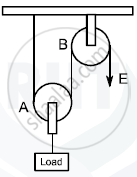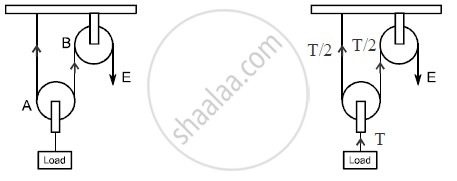# The Diagram Below Shows a Pulley Arrangement : (I) Copy the Diagram and Mark the Direction of Tension on Each Strand of the String. (Ii) What is the Velocity Ratio of the Arrangement ? - Physics

The diagram below shows a pulley arrangement :(i) Copy the diagram and mark the direction of tension on each strand of the string.
(ii) What is the velocity ratio of the arrangement ?
(iii) If the tension acting on the string is T, then what is the relationship between T and effort E ?
(iv) If the free end of the string moves through distance x, find the distance by which the load is raised.

#### Solution

(i)(ii) The load rises to x/2
∴  velocity ratio is 2
(iii) T/2 = E

(iv) x/2

Concept: Pulley
Is there an error in this question or solution?